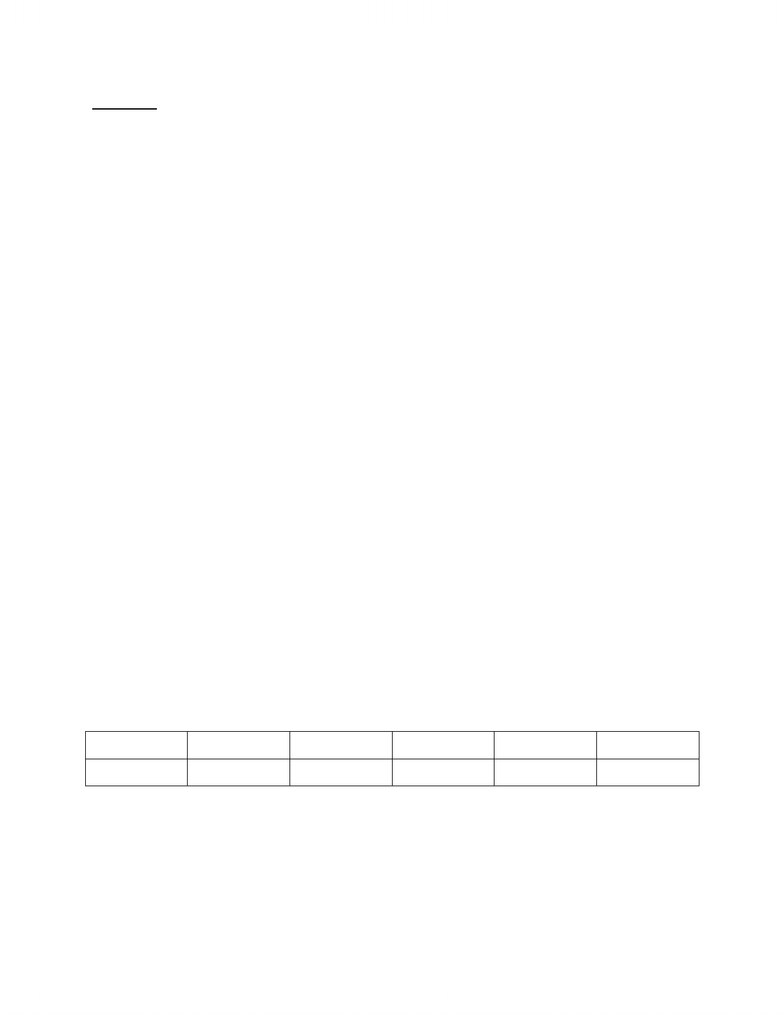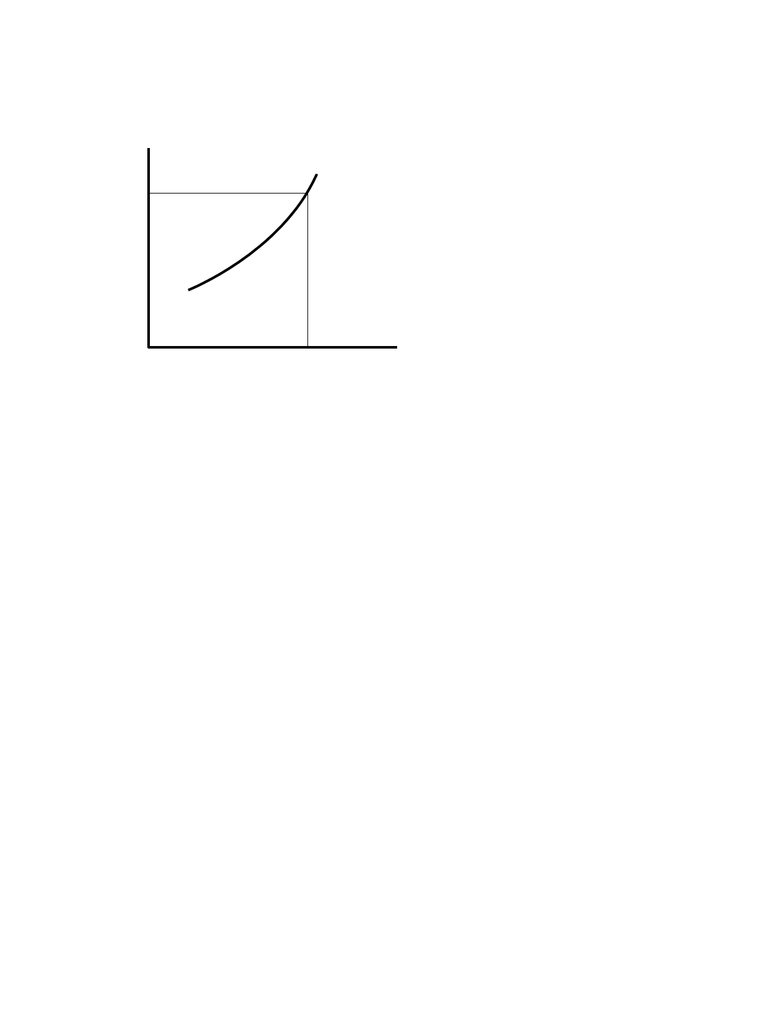Class Notes (1,100,000)
CA (630,000)
U of C (8,000)
BSEN (50)
Lecture

BSEN 401 Lecture Notes - Ceteris Paribus, Opportunity Cost, Economic Equilibrium

Department
Course Code
BSEN 401
Professor
William Huddleston

This preview shows pages 1-2. to view the full 7 pages of the document.SUPPLY:
Definition: Supply is the quantities of goods and services supplied by firms at every price ceteris
paribus
Supply is the relationship between 2 variables, price (P) and quantity supplied (q for the
firm and Q for the industry, the sum of the firms), holding all other variables constant. The most
important of the ceteris paribus conditions are the length of the supply period, the costs of inputs
into production, the prices of other goods (Pi where ‘i’ represents one of n commodities), and
technology. We can express the Supply for the x commodity as
S(qX or QX): qX or QX = f(PX Inputs Costs, Pi, Time Period, Technology)
Note: Supply is defined for quantity supplied in response to price not vice versa. Supply is
therefore a competitive concept so that monopolies, for example, do not have supply
functions.
Law of Supply: Quantity Supplied is positively related to Price
Constant input costs imply that an increase in price will increase profit and thus quantity
supplied. The quantity supplied only increases to the point where price equals opportunity cost.
Analyzing the supply function is a key part of this course but we will leave it until later.
Example: The following table shows the number of kilograms of ground beef supplied by a firm
during a year to given prices of ground beef, ceteris paribus.
Price
\$5
\$4
\$3
\$2
\$1
Quantity
40
15
25
10
0

Only pages 1-2 are available for preview. Some parts have been intentionally blurred.We graph this relation with Price on the vertical axis and quantity on the horizontal axis.
Note: A change in the price of the commodity implies a change in quantity supplied but not a
change in supply. A change in a ceteris paribus condition implies a change in supply.
Changes (shifts) in Supply:
1. A change in Per Unit Costs
Supply gives us the amount that a firm would produce at a given price so Supply is
essentially determined by the firm’s costs. A change in per unit costs changes the cost of
producing a unit of output and thus changes the amount that a firm will produce. An increase in
costs per unit of output means that the firm has to charge more to cover costs; supply therefore
shifts up by the amount of the increase in per unit costs. We say that supply decreases in this case
because the firm decreases quantity supplied at any given price.
Example. The graph below shows the fall in supply due to an increase in costs of \$1 per unit.
Price (\$s)
Quantity (kilos)
1
2
5
3
4
60
30
10 20 40 50
So
You're Reading a Preview

Unlock to view full version